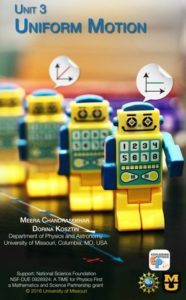eUnit 3 Uniform MotionThrough an engaging lesson, students observe and describe the motion of cars. Utilizing bubble tubes, students study uniform motion: measure the position of the bubble as a function of time, build a position-time graph, interpret its slope and calculate the speed of the bubble. They also learn to convert non-standard units to metric units. To reinforce concepts of position, distance, and change in position, students use a battery-operated car to develop motion diagrams. Students utilize technology to understand the difference between speed and velocity. By the end of the unit, students will be able to answer the following questions:

• How do you describe the motion of an object?
• How do you measure speed?
• How do you pictorially represent uniform motion?
• How do you mathematically calculate the factors involved in uniform motion?
• How do you calculate average speed?
• How do you predict where the paths of two objects traveling at a constant speed intersect?

Timeline for teaching Unit 3 (draft)

Big Ideas
1. Uniform motion means that an object travels equal distance in equal time intervals.
2. Position, distance and displacement have different meanings.
3. Motion can be described in different ways: with words, graphs, motion diagrams and mathematical models.

Learning Goals and Objectives
By the end of this unit, the students should be able to:

1. From a conducted experiment of an object in uniform motion, analyze the position, time and velocity of the object. (DOK4) a) Distinguish between position, change in position, and distance. b) Distinguish between an instant in time and a time interval. c) Describe the motion of an object, including the starting position, direction of motion, time elapsed, and velocity. d) Draw an x vs. t graph and a v vs. t graph for an object’s motion. e) Graphically determine the average velocity of an object from the slope of an x vs. t graph. f) Use technology (i.e., motion detector) to collect and analyze uniform motion data. g) Analyze and compare the motion of two objects.
2. Describe an object in uniform motion using multiple representations (i.e., verbal descriptions, motion diagrams, graphs and mathematical models). (DOK3) a) Verbally describe the motion of an object in uniform motion. b) Draw a motion diagram for an object in uniform motion. c) Construct x vs. t and v vs. t graphs and interpret data. d) Mathematically describe uniform motion as the slope of an x vs. t graph. e) Convert among verbal descriptions, motion diagrams, graphs and mathematical models.
3. Mathematically and graphically determine the displacement and velocity of an object in uniform motion. (DOK3) a) Construct the corresponding v vs. t graph from a given x vs. t graph. b) Construct the corresponding x vs. t graph from a given v vs. t graph. c) Compare and contrast x vs. t graphs with different slopes. d) Use the mathematical expression to calculate speed, displacement or time interval of an object in uniform motion. e) Determine the displacement of an object by finding the area under the curve of a v vs. t.
4. Calculate the average speed for an object in uniform motion.(DOK3) a) Analyze the motion of a segmented x vs. t or v vs. t graph. b) Determine the average speed of an object by using the equation:???
5. Design and conduct experiments to study uniform motion. (DOK4) a) Make observations. b) Operationally define the variables. c) Generate a hypothesis. d) List the steps of the procedure used to collect the data. e) Collect the data and organize it into a table. f) Convert metric units as needed. g) Graph and interpret the results. h) Develop conclusions based on results.